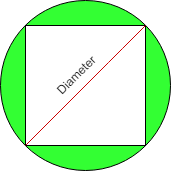# Area of square Circumscribed by Circle

• Last Updated : 07 Nov, 2021

Given the radius(r) of circle then find the area of square which is Circumscribed by circle.
Examples:

```Input : r = 3
Output :Area of square = 18

Input :r = 6
Output :Area of square = 72```

Attention reader! All those who say programming isn't for kids, just haven't met the right mentors yet. Join the  Demo Class for First Step to Coding Coursespecifically designed for students of class 8 to 12.

The students will get to learn more about the world of programming in these free classes which will definitely help them in making a wise career choice in the future.

All four sides of a square are of equal length and all four angles are 90 degree. The circle is circumscribed on a given square shown by a shaded region in the below diagram.Properties of Circumscribed circle are as follows:

• The center of the circumcircle is the point where the two diagonals of a square meet.
• Circumscribed circle of a square is made through the four vertices of a square.
• The radius of a circumcircle of a square is equal to the radius of a square.

Formula used to calculate the area of circumscribed square is:
2 * r2
where, r is the radius of the circle in which a square is circumscribed by circle.
How does this formula work?
Assume diagonal of square is d and length of side is a.
We know from the Pythagoras Theorem, the diagonal of a
square is √(2) times the length of a side.
i.e d2 = a2 + a2
d = 2 * a2
d = √(2) * a
Now,
a = d / √2
and We know diagonal of square that are Circumscribed by
Circle is equal to Diameter of circle.
so Area of square = a * a
= d / √(2) * d / √(2)
= d2/ 2
= ( 2 * r )2/ 2 ( We know d = 2 * r )
= 2 * r2

## CPP

 `// C++ program to find Area of``// square Circumscribed by Circle``#include ``using` `namespace` `std;`` ` `// Function to find area of square``int` `find_Area(``int` `r)``{``    ``return` `(2 * r * r);``}`` ` `// Driver code``int` `main() ``{``    ``// Radius of a circle``    ``int` `r = 3;``     ` `    ``// Call Function to find ``    ``// an area of square``    ``cout << ``" Area of square = "` `                 ``<< find_Area(r);``     ` `    ``return` `0;``}`

## Java

 `// Java program to find Area of``// square Circumscribed by Circle``class` `GFG {``     ` `    ``// Function to find area of square``    ``static` `int` `find_Area(``int` `r)``    ``{``        ``return` `(``2` `* r * r);``    ``}``     ` `    ``// Driver code``    ``public` `static` `void` `main(String[] args)``    ``{``        ``// Radius of a circle``        ``int` `r = ``3``;`` ` `        ``// Call Function to find``        ``// an area of square``        ``System.out.print(``" Area of square = "``                             ``+ find_Area(r));``    ``}``}`` ` `// This code is contributed by Anant Agarwal.`

## Python3

 `# Python program to``# find Area of``# square Circumscribed``# by Circle`` ` `# Function to find``# area of square``def` `find_Area(r):`` ` `    ``return` `(``2` `*` `r ``*` `r)``     ` `# driver code``# Radius of a circle``r ``=` `3``     ` `# Call Function to find ``# an area of square``print``(``" Area of square = "``, find_Area(r))`` ` `# This code is contributed``# by Anant Agarwal.`

## C#

 `// C# program to find Area of``// square Circumscribed by Circle``using` `System;`` ` `class` `GFG {``     ` `    ``// Function to find area of square``    ``static` `int` `find_Area(``int` `r)``    ``{``        ``return` `(2 * r * r);``    ``}``     ` `    ``// Driver code``    ``public` `static` `void` `Main()``    ``{``        ``// Radius of a circle``        ``int` `r = 3;`` ` `        ``// Call Function to find``        ``// an area of square``        ``Console.WriteLine(``" Area of square = "``                            ``+ find_Area(r));``    ``}``}`` ` `// This code is contributed by vt_m.`

## PHP

 ``

## Javascript

 ``

Output:

`Area of square = 18`

Time Complexity: O(1)

My Personal Notes arrow_drop_up